# Themeparkpatterns
Fractions and Decimals

A fraction is an expression representing a part or several equal parts of a unit. Fractions can also be expressed in decimal form.

Places To GoPeople To SeeThings To DoTeacher ResourcesBibliographyPlaces To Go

The following are places to go (some real and some virtual) to find out about fractions and decimals.

Baseball Math-Simplifying Fractions Game
Travel to a virtual baseball diamond to hone your skills of simplifying fractions.
Computation Castle
Travel to the Computation Castle to learn about equivalent and improper fractions.
Egyptian Fractions
Visit ancient Egypt and learn about their system of fractions. Have students discuss if the Egyptian method of working with fractions is more effective or efficient than what we do today.
Fractions
This site contains information, graphics and quizzes on basic concepts of fractions.
Soccer Math- Multiplying Fractions Game
Practice multiplying fractions at a virtual math soccer field.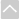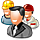People To See

See the experts at AAA Math to practice your fraction skills online.
Dr. Math
Get to know Dr. Math and send him your questions about fractions. This portion of his site is from his archived fraction and decimals page and contains previously asked questions and answers.
EZ Fractions
Hang out with Mr. Nussbaum. He has a variety of ways to hone your fraction and decimal skills.
Fractions Decimals Percents Jeopardy Game
Meet a virtual Alex Trebek and play a fraction and decimal Jeopardy game.
Melvil Dewey: Founder of Book Classification
Meet Melvil Dewey. Nobody knows decimals like Dewey.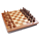Things To Do

Practice adding, comparing, converting, dividing, multiplying, reducing, and subtracting fractions.
Complex Fractions
A complex fraction is a fraction where the numerator, denominator, or both contain a fraction. This is part of the great SOS Math site.
Compound Fractions
A compound fraction is sometimes called a mixed number. This is part of the great SOS Math site.
Converting Decimals to Fractions
Find simple explanations and good illustrations of fractions concepts. This is part of the great SOS Math site.
Decimals, Whole Numbers, and Exponents
Find everything you ever wanted to know about decimals.
Fraction to Decimal Conversion Tables
Does 1/5 = 0.2, 0.4 or 0.6? Learn how to easily convert fractions to decimals.
Fractions
Changing improper fractions to mixed numbers is just one of a dozen fractions topics that you can brush up on at this site.
Free Worksheets
Find a variety of free, printable math worksheets. This math page is part of the larger Free Worksheets site that has worksheets in the areas of Language Arts, Science, Geography, Reading, Thinking Skills, History, etc.
FunBrain: Freshed Baked Fractions
Fresh Baked Fractions is a game to practice simplifying fractions.
Illuminations Interactive Fraction Tool
Use this interactive one-person game to practice your skills while you explore relationships among fractions and ways of combining fractions.
No Matter What Shape Your Fractions are In
Determine fraction equivalents from geometric shapes.
Simple Fractions
This review format will guide you easily through all operations involving simple fractions. The review will include definitions, rules, worked examples, problems, typical mistakes, answers, and step-by-step solutions. This is part of the great SOS Math site.
Visual Fractions
A fractions tutorial that models fractions with number lines or circles. Fraction games are included.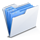Teacher Resources

Lesson Plans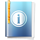Bibliography

• Adler, David A. Fraction Fun. New York: Holiday House, c1996.
• Bloomberg, Jerome. Multiplication and Division of Decimals. New York : Harper's College Press, 1975.
• Cummings, Alyece B. Painless Fractions. Hauppauge, N.Y.: Barron's, c1998.
• Hein, Marilyn B. Math Phonics. Fractions: Quick Tips and Alternative Techniques for Math Mastery. Carthage, IL: Teaching & Learning Co.; c1998.
• King, Andrew. Making Fractions. Brookfield, Conn.: Copper Beech Books, 1998.
• Leedy, Loreen. Fraction Action. New York: Holiday House, c1994.
• Stienecker, David. Fractions. New York: Benchmark Books, 1996.
• Wood, Merle W. Decimals, Fractions, and Percentages for Job and Personal Use. Cincinnati, Ohio: South-Western Pub. Co., c1992